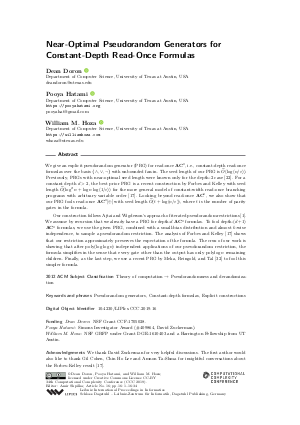Document# Near-Optimal Pseudorandom Generators for Constant-Depth Read-Once Formulas

### Authors Dean Doron, Pooya Hatami, William M. Hoza## File

LIPIcs.CCC.2019.16.pdf
• Filesize: 0.68 MB
• 34 pages

## Acknowledgements

We thank David Zuckerman for very helpful discussions. The first author would also like to thank Gil Cohen, Chin Ho Lee and Amnon Ta-Shma for insightful conversations about the Forbes-Kelley result [Michael A. Forbes and Zander Kelley, 2018].

## Cite As

Dean Doron, Pooya Hatami, and William M. Hoza. Near-Optimal Pseudorandom Generators for Constant-Depth Read-Once Formulas. In 34th Computational Complexity Conference (CCC 2019). Leibniz International Proceedings in Informatics (LIPIcs), Volume 137, pp. 16:1-16:34, Schloss Dagstuhl - Leibniz-Zentrum für Informatik (2019)
https://doi.org/10.4230/LIPIcs.CCC.2019.16

## Abstract

We give an explicit pseudorandom generator (PRG) for read-once AC^0, i.e., constant-depth read-once formulas over the basis {wedge, vee, neg} with unbounded fan-in. The seed length of our PRG is O~(log(n/epsilon)). Previously, PRGs with near-optimal seed length were known only for the depth-2 case [Gopalan et al., 2012]. For a constant depth d > 2, the best prior PRG is a recent construction by Forbes and Kelley with seed length O~(log^2 n + log n log(1/epsilon)) for the more general model of constant-width read-once branching programs with arbitrary variable order [Michael A. Forbes and Zander Kelley, 2018]. Looking beyond read-once AC^0, we also show that our PRG fools read-once AC^0[oplus] with seed length O~(t + log(n/epsilon)), where t is the number of parity gates in the formula. Our construction follows Ajtai and Wigderson’s approach of iterated pseudorandom restrictions [Ajtai and Wigderson, 1989]. We assume by recursion that we already have a PRG for depth-d AC^0 formulas. To fool depth-(d + 1) AC^0 formulas, we use the given PRG, combined with a small-bias distribution and almost k-wise independence, to sample a pseudorandom restriction. The analysis of Forbes and Kelley [Michael A. Forbes and Zander Kelley, 2018] shows that our restriction approximately preserves the expectation of the formula. The crux of our work is showing that after poly(log log n) independent applications of our pseudorandom restriction, the formula simplifies in the sense that every gate other than the output has only polylog n remaining children. Finally, as the last step, we use a recent PRG by Meka, Reingold, and Tal [Meka et al., 2019] to fool this simpler formula.

## Subject Classification

##### ACM Subject Classification
• Theory of computation → Pseudorandomness and derandomization
##### Keywords
• Pseudorandom generators
• Constant-depth formulas
• Explicit constructions

## Metrics

• Access Statistics
• Total Accesses (updated on a weekly basis)
0